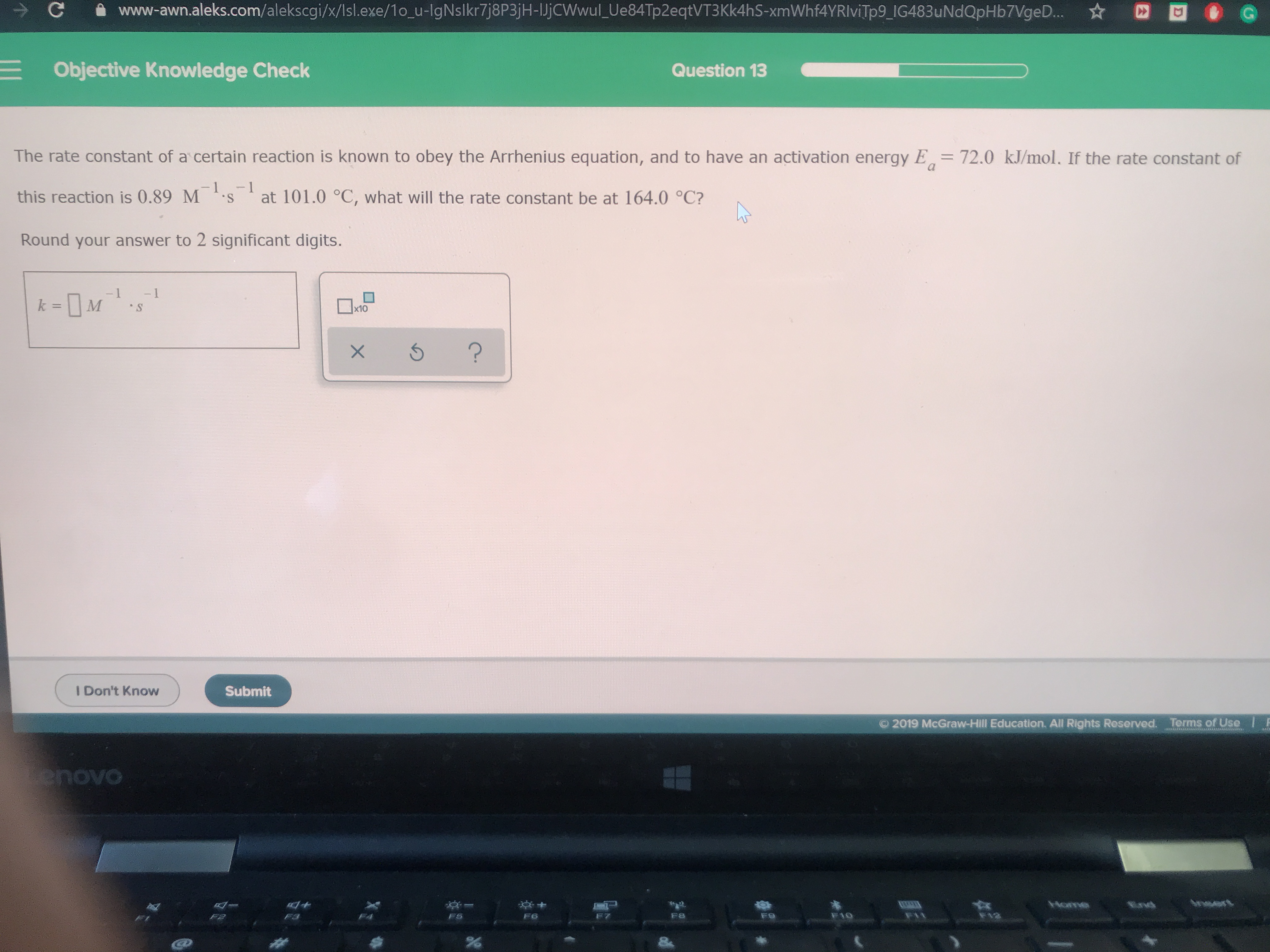# The rate constant of a certain reaction is known to obey the Arrhenius equation and to have an activation energy, Ea = 7.20 kJ/mol. If the rate constant of this reaction is 0.89 M^-1*^s-1 at 101.0 deg C. What will the rate constant be at 164.0 deg C?